Function Repository Resource:

# FunctionPeriodPlot

Plot a given number of periods of a periodic function

Contributed by: Wolfram|Alpha Math Team
 ResourceFunction["FunctionPeriodPlot"][f,x] plots one period of a periodic function f of x. ResourceFunction["FunctionPeriodPlot"][f,x,n] plots n periods of a periodic function f of x.

## Details and Options

The function to plot, f, is expected to be periodic in the variable x.
 "PeriodAlignment" Center determine where to align the period relative to the starting point using the values Left,Right or Center "StartingPoint" 0 where to start plotting period
ResourceFunction["FunctionPeriodPlot"] also takes all options of Plot.
The options "StartingPoint" and "PeriodAlignment" work in tandem to determine the plot.

## Examples

### Basic Examples (1)

Plot a single period of a periodic function:

 In:=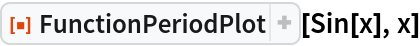Out=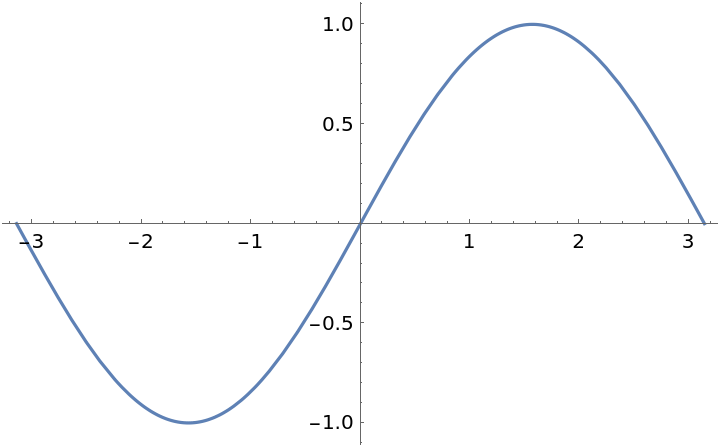### Scope (1)

Plot two periods of a periodic function:

 In:=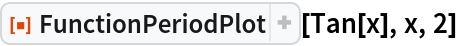Out=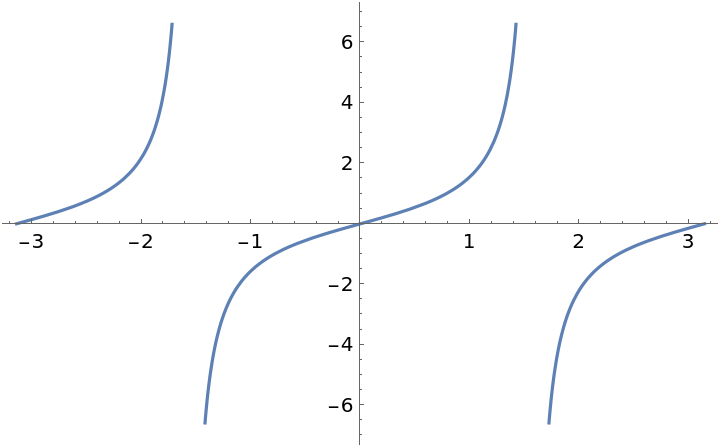### Options (1)

Use "StartingPoint" to determine the base point and "PeriodAlignment" to determine whether to plot the period(s) centered around that point, to the right of that point or to the left of that point:

 In:=Out=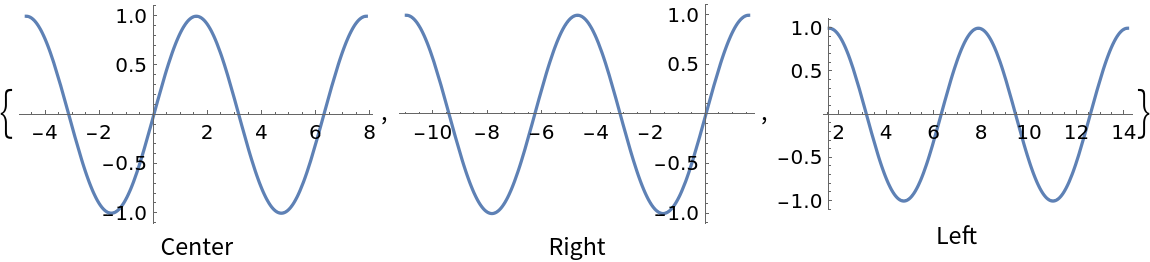### Possible Issues (1)

FunctionPeriodPlot returns unevaluated when the input expression is not periodic:

 In:=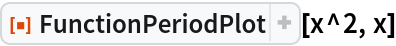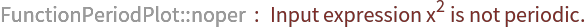Out=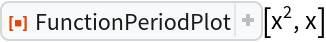## Publisher

Wolfram|Alpha Math Team

## Version History

• 2.0.0 – 23 March 2023
• 1.0.0 – 26 October 2020

## Author Notes

To view the full source code for FunctionPeriodPlot, evaluate the following:

 In:=